# Exploring Data Graphically

Before we start simplifying our data with descriptive statistics and then models, we should always explore all the data. A great way to do that is graphically.

William Murrah www.statistical-thinking.com (QMER)aub.ie/qmer
2020-09-08
library(mosaic)


Before you start simplifying your data with descriptive statistics, it is essential that you explore all your data. A reasoned use of graphics is indispensable for this purpose. In this tutorial, I will demonstrate some of the most commonly used graphical methods to explore experimental data.

R has extensive methods for graphics, which allow for amazing visualizations of data. My purpose here is to teach you the basics of graphics, so I will stick with some relatively simple graphics in base R.

## Bar Graphs

Typically, the type of graph you use depends on the type of data you are exploring.

We will use the mtcars data, which is part of base R. Below, I load the data, then transform two variables to factors, which is how R handles categorical variables. Take a look at the help file for mtcars by typing ?mtcars into the R console. Look at the data description under the “Format” heading to see how I decided to label the two factors vs and am. You should also read through this list of variable names and labels to understand what our data are measuring.

data(mtcars)

mtcars <- transform(mtcars,
vs = factor(vs, labels = c("v-shaped", "straight")),
am = factor(am, labels = c("automatic", "manual")))


When we have a categorical variable, we often want to know how many cases are in each category. In R a categorical variable is called a factor, and the categories within that variable are called levels. Let’s look at the vs factor, which describes the shape of the engine for each car. First, I will simply table this variable:

table(mtcars$vs)   v-shaped straight 18 14  We can see that we have 18 cars with a V-shaped engine and 14 with a straight engine. Next, I will use the table function to create a bar plot. barplot(table(mtcars$vs))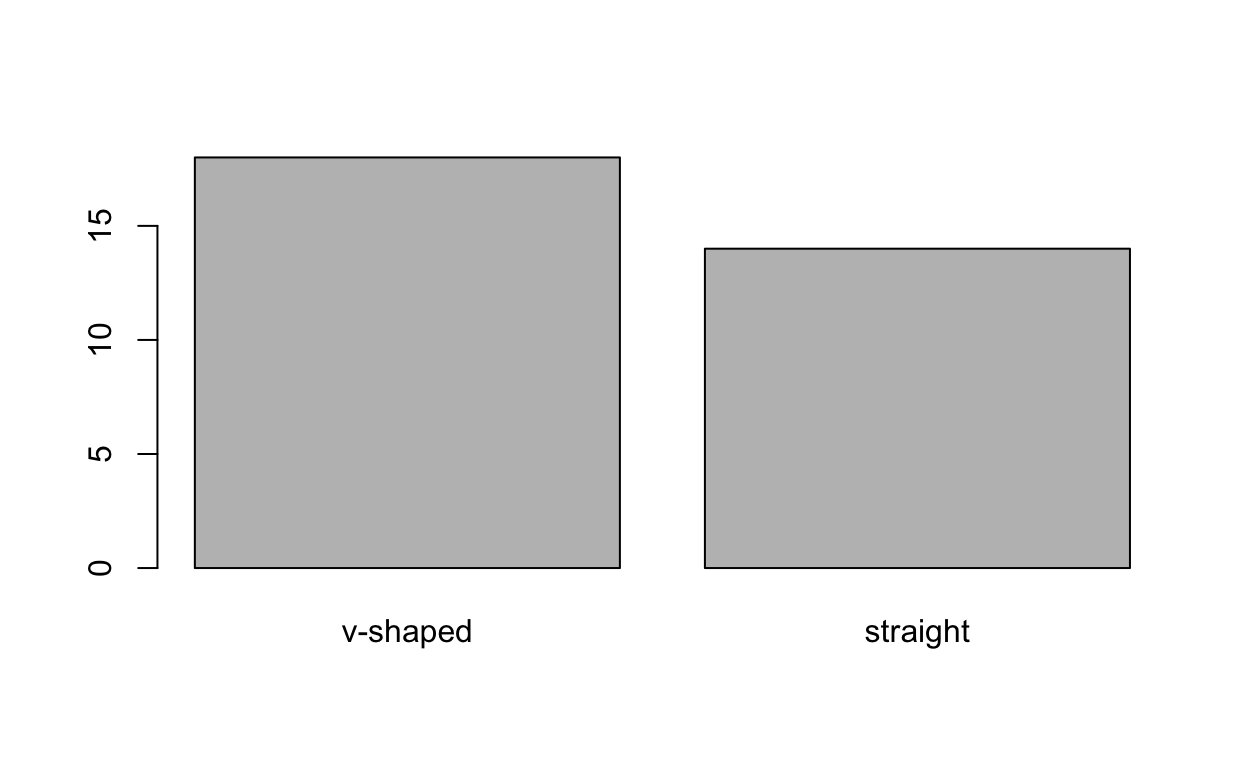Note, that the bar plot needs tabled data, so the table() function is nested within the first argument to this plot.

tab <- table(mtcars$vs, mtcars$am)
colnames(tab)
barplot(tab, beside = TRUE, legend.text = rownames(tab),
main = "Comparing engine shape to transmission type", )


## Histograms

Similar to the bar graph is the histogram. The major difference is that histograms are most often used with continuous variables, instead of categorical variable. While bar graphs have natural groupings (the categories or levels), continuous variables do not. So, to create a histogram a continuous variable is binned into groups and the frequencies of cases in the range of those groups is plotted with a bar.

hist(mtcars$mpg)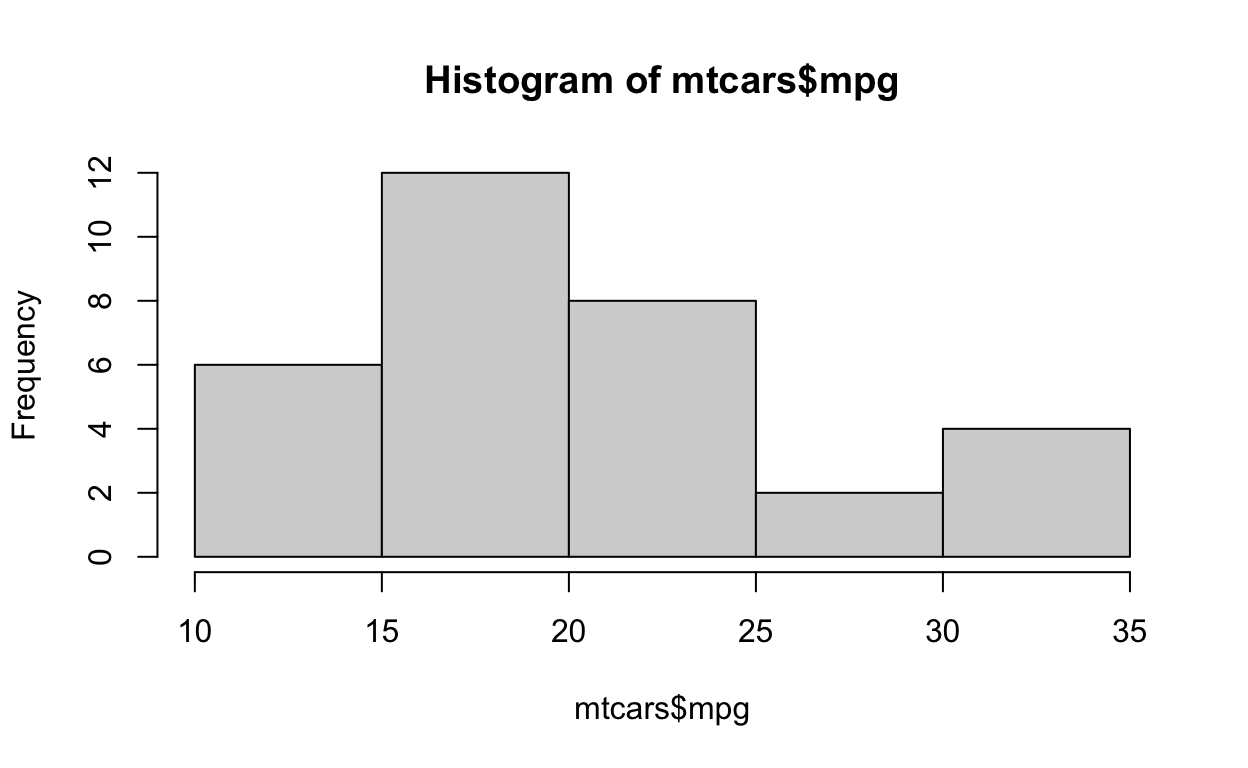It is very important to remember that when you create a histogram a decision is being make about how to bin the data. This can impact the shape of the distribution, and your interpretation. It is often a good idea to play around with this binning. To do that in the R hist() function you can use the breaks = argument. This allows you to set the number of bins. For example, compare the two histograms of mpg. hist(mtcars$mpg, breaks = 3, main = "Histogram pf mpg with 3 breaks")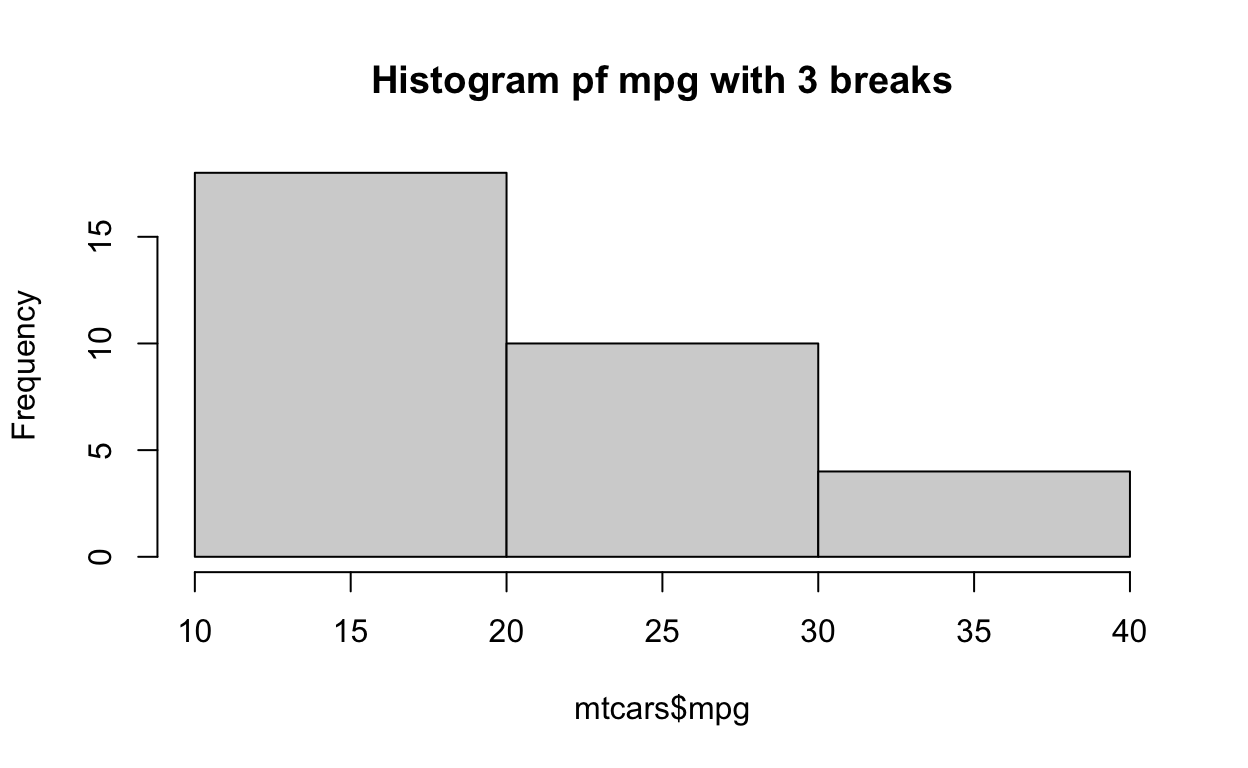hist(mtcars$mpg, breaks = 13, main = "Histogram of mpg with 13 breaks")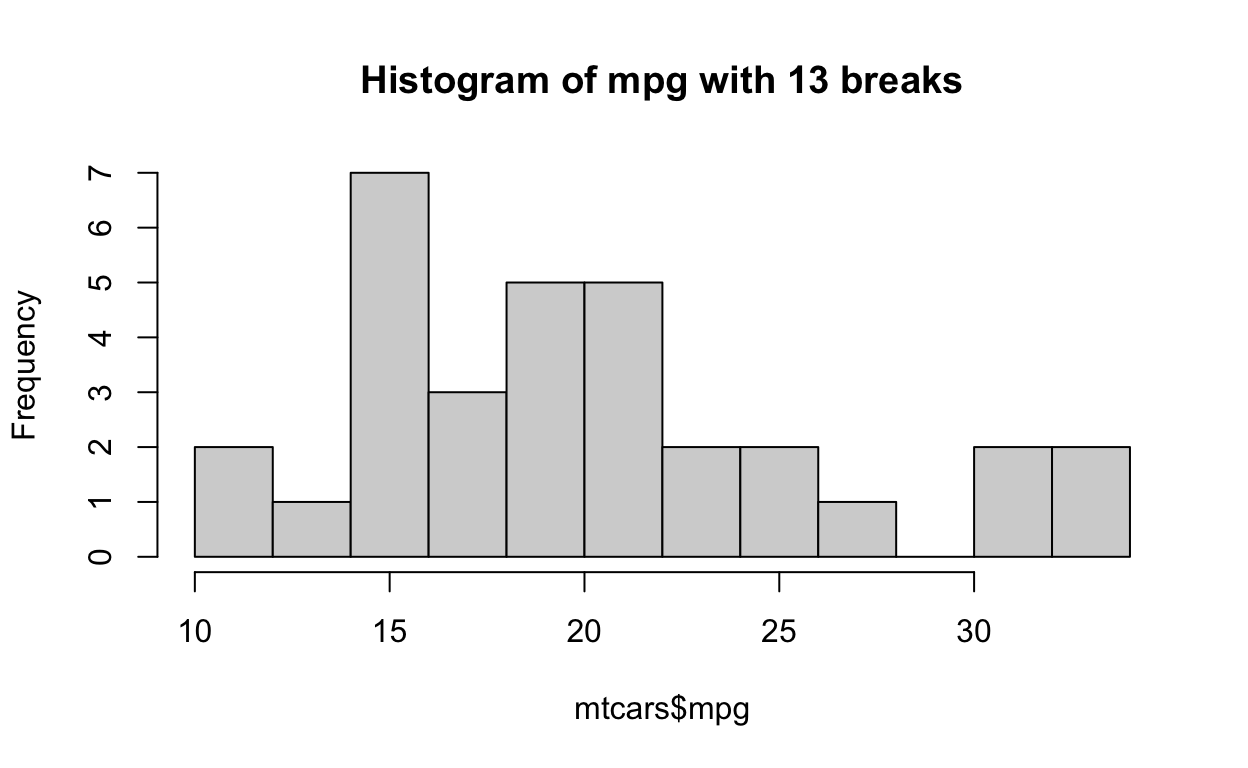Also note that I called for 13 bins, but was given less than that. So, the hist() function only takes this value as a suggestion. See ?hist for more information. plot(density(mtcars$mpg))


## Boxplots

Boxplots or box and whisker plots, are also useful for exploring the distribution of continuous variables. These plots visualize the median, interquartile range, the full range, and look for extreme values.

boxplot(mtcars$mpg)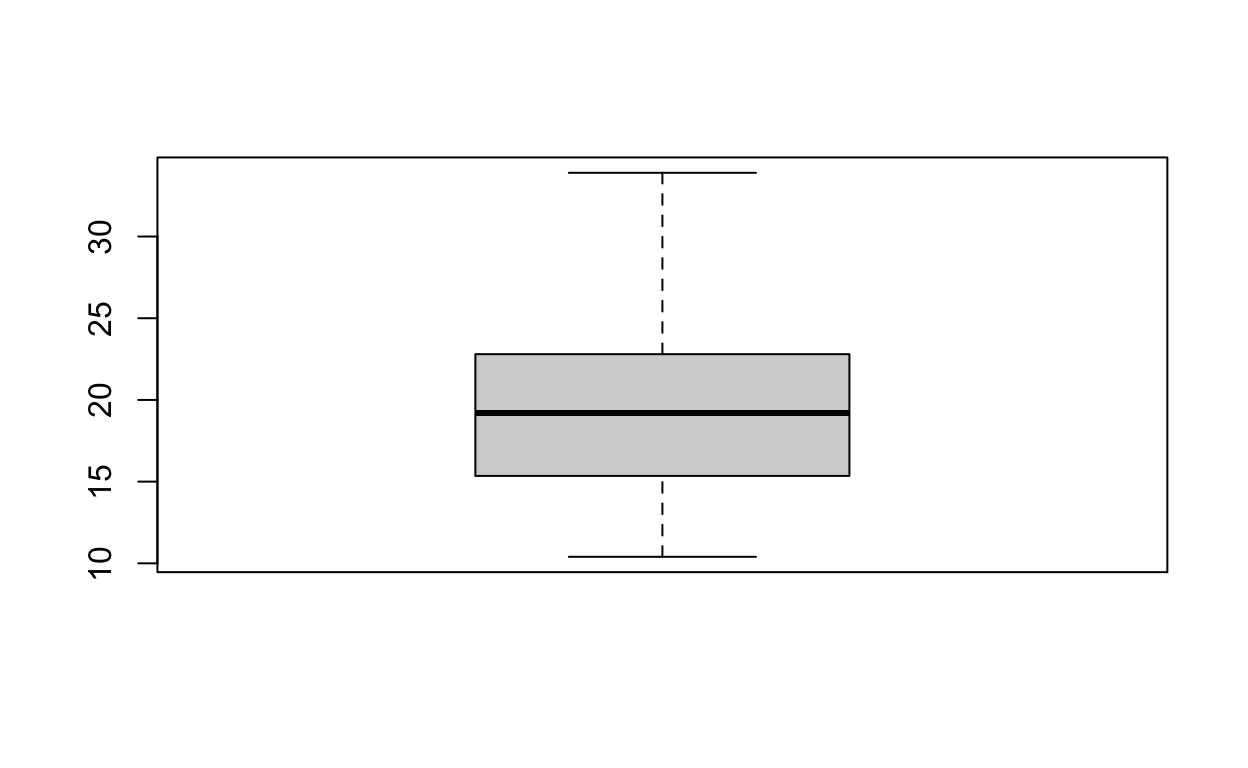(qtle <- quantile(mtcars$mpg))

    0%    25%    50%    75%   100%
10.400 15.425 19.200 22.800 33.900 
boxplot(mtcars$mpg, horizontal = TRUE) text(x = qtle, y = 1.3, labels = paste("0%\n", qtle)) text(x = qtle, y = 1.3, labels = paste("25%\n", qtle)) text(x = qtle, y = 1.3, labels = paste("50%\n", qtle)) text(x = qtle, y = 1.3, labels = paste("75%\n", qtle)) text(x = qtle, y = 1.3, labels = paste("100%\n", qtle))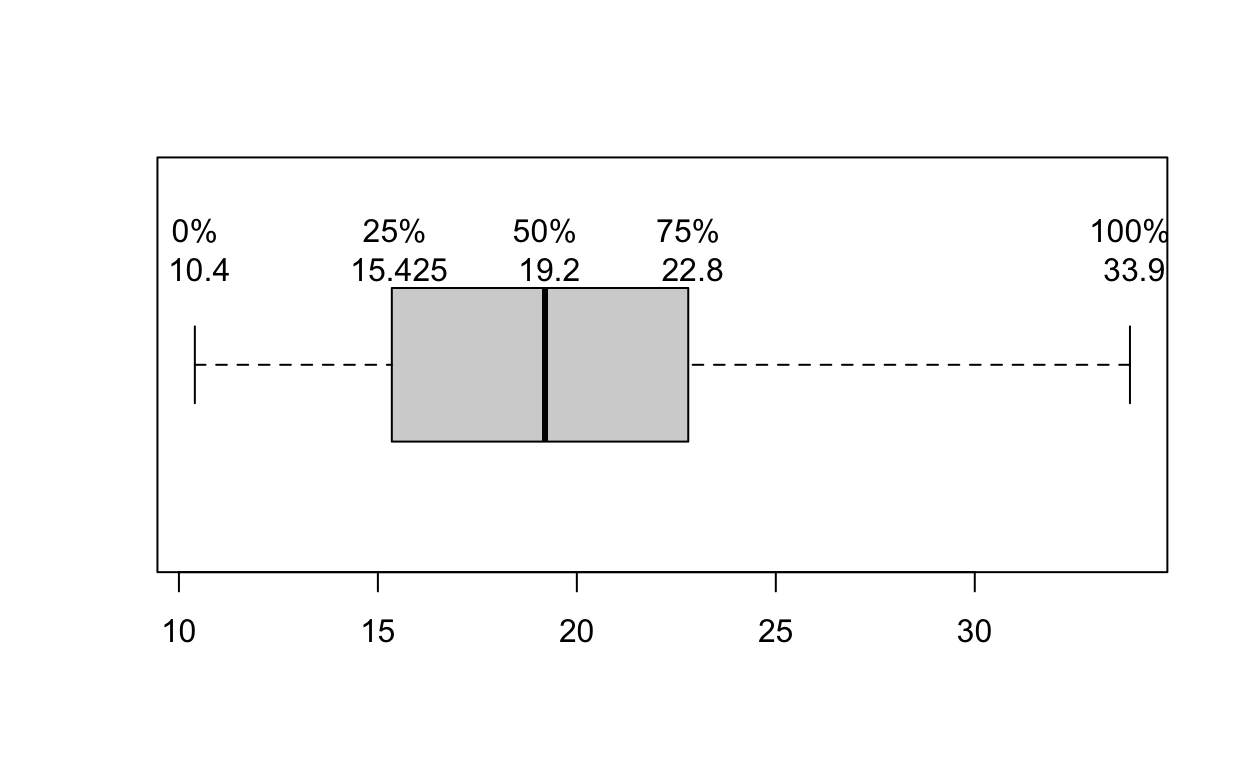hist(mtcars$mpg)boxplot(mpg ~ vs, data = mtcars)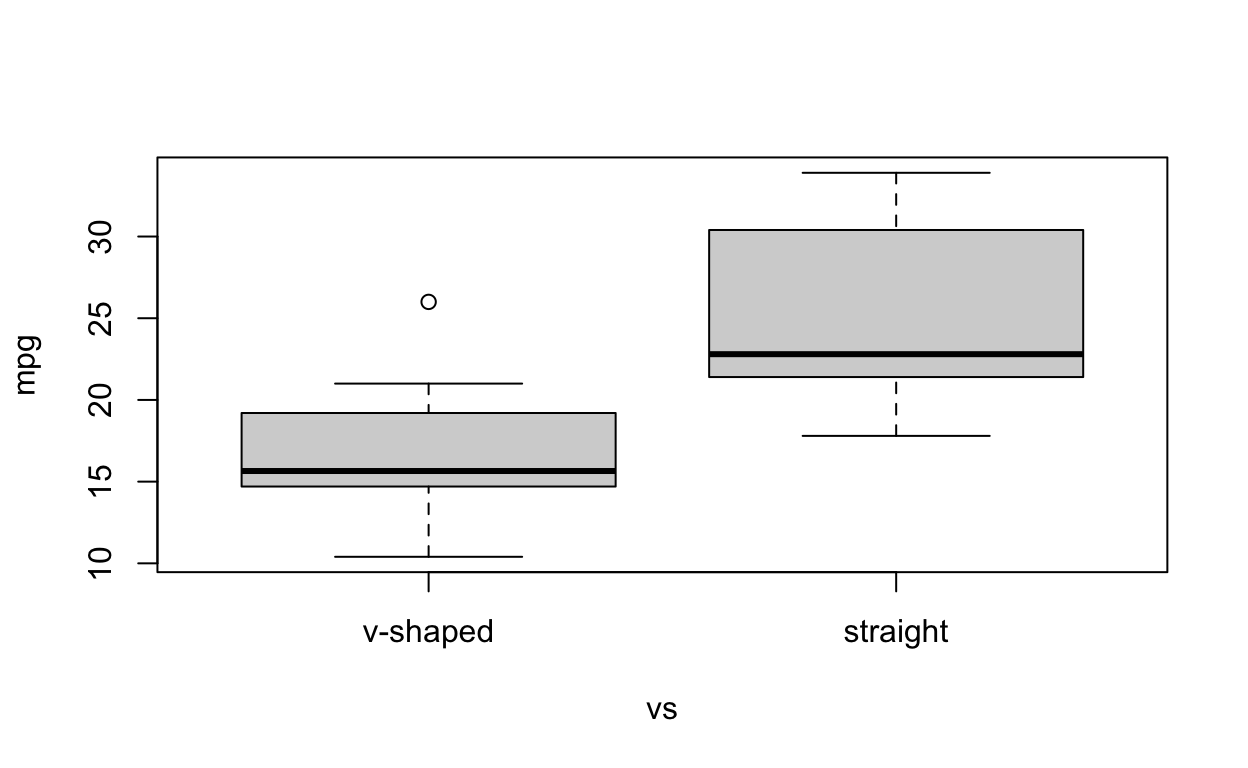boxplot(mpg ~ vs * am, data = mtcars, las = 3, ann = FALSE, xaxt = "n")
title(ylab = "Miles per Gallon",
xlab = "Engine Shape and Transmission Type",
main = "Miles per Gallon by Engine Shape and Transmission Type")
axis(side = 1, at = 1:4, mgp = c(1,2,0),
labels =c("V-shaped\nAutomatic", "straight\nAutomatic",
"V-shaped\nManual", "straight\nManual"))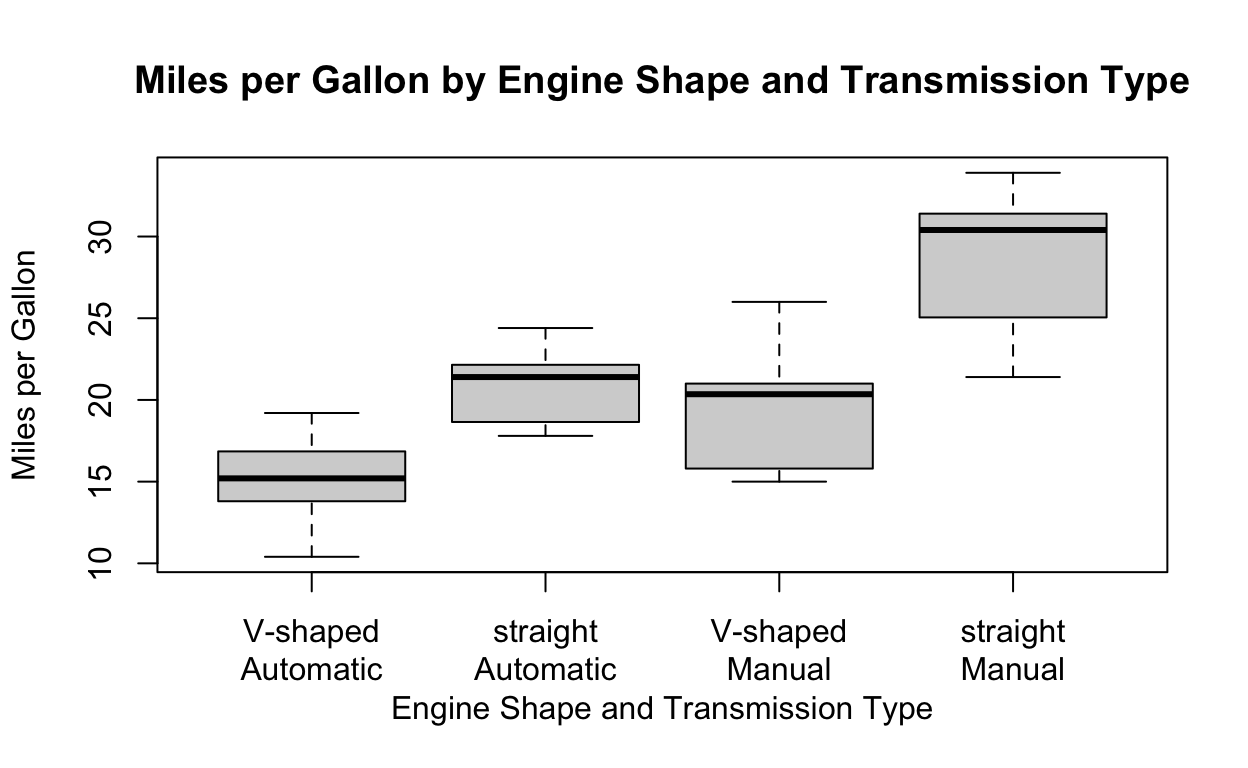## Scatterplots

Scatterplots are useful when we want to compare two numeric variables. For example, let’s look at the relation between miles per gallon and horsepower.

plot(mpg ~ hp, data = mtcars)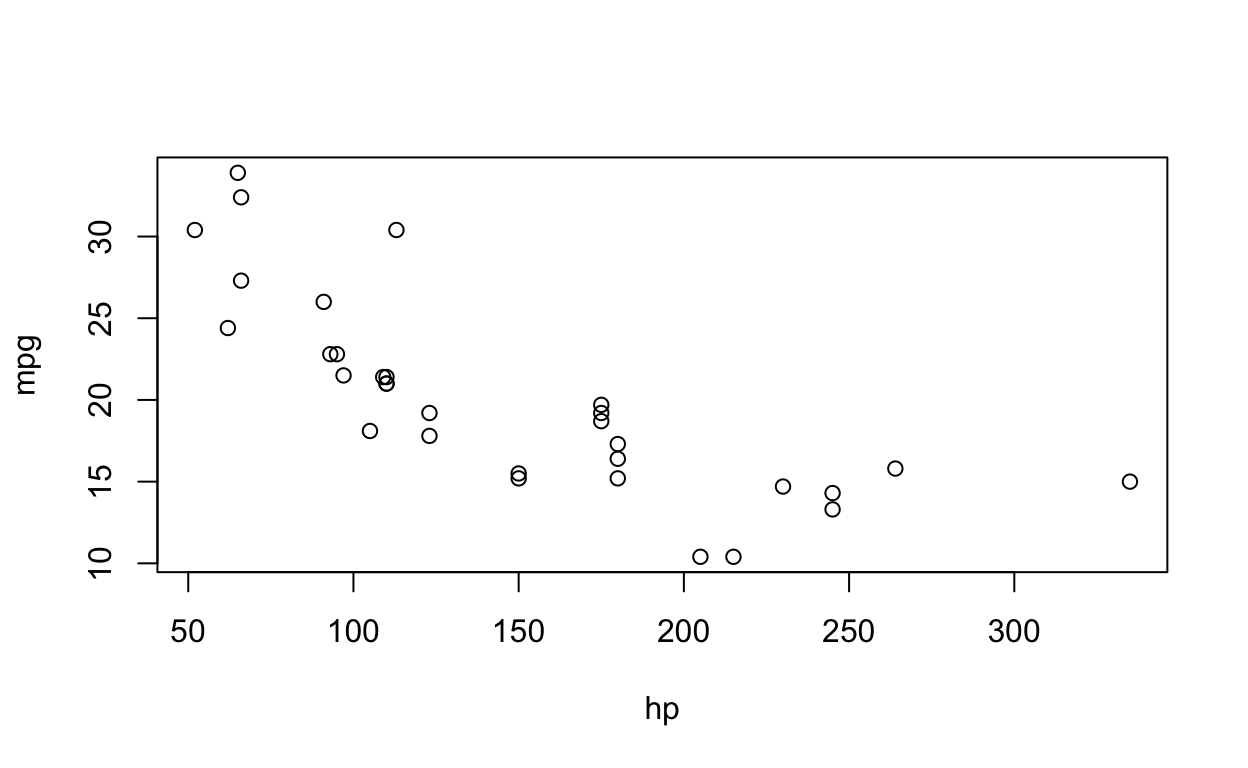Note that each point on the plot represents a single car, and indicates it’s horsepower on the x axis and it’s miles per gallon on the y axis. For example, the car represented by the left most point has a hp of about 50 and a mpg of a little more than 30. We see a negative relation between these two variables, a car with more horsepower is likely to have a lower miles per gallon.